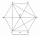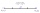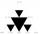# Mean + line segment - math problems

the arithmetic mean (simply the mean or average) is the sum of a collection of numbers divided by the count of numbers in the collection. The arithmetic mean is the most commonly used and readily understood measure of central tendency in a data set. It is a statistical characteristic of the center of a given data set.

Arithmetic mean is a quantity very sensitive to extreme values, so the median and mode are also used in practice. Mode is the most common value. The median halves the ordered file.

#### Number of problems found: 9

• My fatherMy father cut 78 slats on the fence. The shortest of them was 97 cm long, the longer one was 102 cm long. What was the total length of the slats in cm?
• Coordinates hexagonThe regular hexagon ABCDEF is given. Point A has coordinates [1; 3] and point D has coordinates [4; 7]. Calculate the sum of the coordinates of the center of its described circle.
• Coordinates of a centroindLet’s A = [3, 2, 0], B = [1, -2, 4] and C = [1, 1, 1] be 3 points in space. Calculate the coordinates of the centroid of △ABC (the intersection of the medians).
• MG=7x-15,MG=7x-15, FG=33, x=? Point M is the midpoint of FG. Find unknown x.
• Midpoint 6FM=8a+1, FG=42, a=? Point M is the midpoint of FG. Find unknown a.
• Find midpointFM=5y+13, MG=5-3y, FG=? M is the midpoint of FG. Use the given information to find the missing measure or value.
• Midpoint 4If the midpoint of a segment is (6,3) and the other end point is (8,-4) what are thw coordinate of the other end?
• Find the 3Find the distance and mid-point between A(1,2) and B(5,5).
• Triangle midpointsDetermine coordinates of triangle ABC vertices if we know tirangle sides midpoints SAB [0;3] SBC [1;6] SAC [4;5], its sides AB, BC, AC.

We apologize, but in this category are not a lot of examples.
Do you have an interesting mathematical word problem that you can't solve it? Submit a math problem, and we can try to solve it.

We will send a solution to your e-mail address. Solved examples are also published here. Please enter the e-mail correctly and check whether you don't have a full mailbox.

Please do not submit problems from current active competitions such as Mathematical Olympiad, correspondence seminars etc...